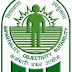Hello Friends,

Today (29 August 2016) Day 3 SSC Has conducted SSC CGL (2016) Exam. In this post we are sharing some of the questions asked in this exam at Evening shift.

1. What element causes radioactive pollution in Kerala?
2. What causes water rain in North Western India?  Ans- Western disturbance in wind
3. Which state has the least population?
4. Washing machine is based on what concept?
5. Father of renaissance in India?  Ans- Raja Ram Mohan Roy
6. Food conduction in plant is through? Ans_ Phloem
7. Headquarter of SAARC?  Ans- Kathmandu, Nepal
8. Who is present Nepal P.M? Ans- Pushpa Kamal Dahal
9. Outermost Range of Himalayas? Ans- Shivalik
10. Sahitya Academy awards are given in how many languages? Ans- 24
11. Which is not an external storage memory?
12. Which part of the brain is associated with hearing?
13. Which of these are soluble in water?
14. Freedom of privacy is related to which article? Ans- Under Article 21

Maths Questions Asked on 29-08-2016 (Evening Shift)

1. The sum of a number and it's reciprocal is 2. Find the number
Options : 1,0,-1, none

2. A train crosses a 50 m long platform in 14 seconds and a pole in 10 seconds find speed of train in Kmph

3. Two ships are sailing in a river on opposite side of a tower having height of 100 m. The angle of elevations for both the shifts are 30° and 60°. Find the distance between the ship. (Exact statement yaad nahi hai jo yaad hai bata diya)

4) 9x^2+16^y2=60
3x+4y=10
Find xy

5) Rs. 2000 amounts to Rs. 4000 in two years on CI. In how much time will it be Rs. 8000?.
6 years
8 years
4 years
None

6) x^2+1/x^2=2 find x-1/x

7) A completes work in 15 days and B In 20 days. They agree to do the work together for Rs. 450. Find share of A

8)In triangle ABC, AD is the median. O is a point on AD which is the centroid. Ratio AO:OD is?

9) Cos@/(1+sin@)+sin@/(1-sin@)=4
Find @
Ans 60°

10) Avg salary of 19 employees is 40,000. If one more employee joins them the avg salary becomes 44000. Find that newly joined employee's salary

11)  If the CP of an article is decreased by 10%. How much should new CP be increased so that the profit remains same

12) Cost of a piece of cloth is Rs. 32 per metre. A shopkeeper announces 25% discount. If any customer wants to have benefit of Rs. 40 then what length of the cloth he should purchase?

13) Ratio of investments made by A and B is 1/3:1/5. They together invested Rs. 960. Find rhe share of each

14) Area of rectangle is 60 sq m and its perimeter is 34. Find the length of its diagonal

15) In a circle a chord AB is drawn O is the center of the circle Radius of circle is 10 cm Length of Chord AB is 16 cm. Find length of OD, where OD is a perpendicular on the chord AB

Online Coaching (Use Coupon Code "QMATHS" to get 25-40% Discount)

SSC CGL 2016 Tier-1 Questions asked on 29-08-2016 (Evening shift)Reviewed by Admin on 10:04:00 PM Rating: 5